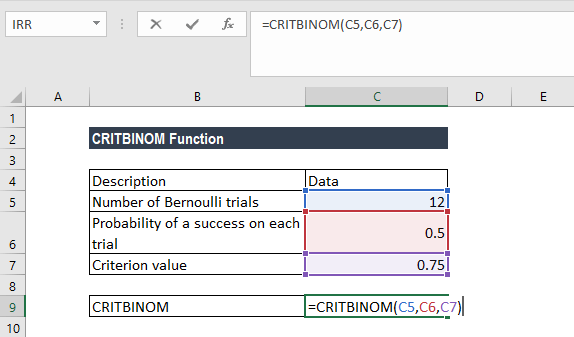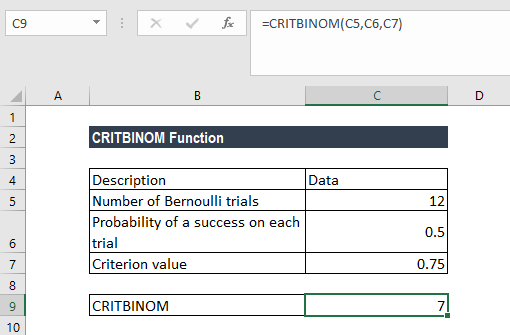# CRITBINOM Function

Returns the smallest value that is greater than or equal to the criterion value in a Cumulative Binomial Distribution

## What is the CRITBINOM Function?

The CRITBINOM function is categorized under Excel Statistical functions. It will return the smallest value that is greater than or equal to the criterion value.

The function returns the inverse of the Cumulative Binomial Distribution. That is, for a specific number of independent trials, CRITBITNOM will return the smallest value (the number of successes) for which the cumulative binomial distribution is greater than or equal to a specified value.

As a financial analyst, CRITBITNOM is useful in data analysis. For example, we can use the function to estimate the highest number of defective products allowed to come off an assembly line run without rejecting the entire lot.

In MS Excel 2010, the BINOM.INV function replaced the CRITBINOM function. However, CRITBITNOM is still stored in the list of compatibility functions to allow compatibility with earlier versions of Excel.

### Formula

=CRITBINOM(trials,probability_s,alpha)

The CRITBITNOM uses the following arguments:

1. Trials (required argument) – This is the number of independent trials to be done. MS Excel will truncate a decimal to an integer.
2. Probability_s (required argument) – This is the probability of success in each trial.
3. Alpha (required argument) – The criterion value of the Cumulative Binomial Distribution (must be between 0 and 1).

### How to use the CRITBINOM Function in Excel?

To understand the uses of the CRITBITNOM function, let’s consider an example:

#### Example

Suppose we are given the following data:

• Number of Bernoulli trials: 12
• Probability of success in each trial: 0.5
• Criterion value: 0.75

The formula to use is:We get the result below:So, the smallest value for which the cumulative binomial distribution is greater than or equal to a criterion value is 7.

### A few notes about the CRITBINOM Function

1. #VALUE! error – Occurs if any of the given arguments is non-numeric.
2. If the trials argument is not an integer, it is truncated.
3. #NUM! error – Occurs when either:
• The given trial argument is less than zero; or
• The given probability_s argument is < 0 or > 1; or
• The given alpha argument is < 0 or > 1.

Thanks for reading CFI’s guide to important Excel functions! By taking the time to learn and master these functions, you’ll significantly speed up your financial analysis. To learn more, check out these additional CFI resources:

• Excel Functions for Finance